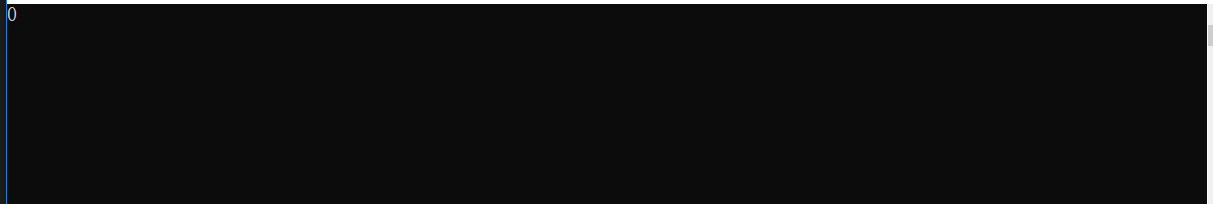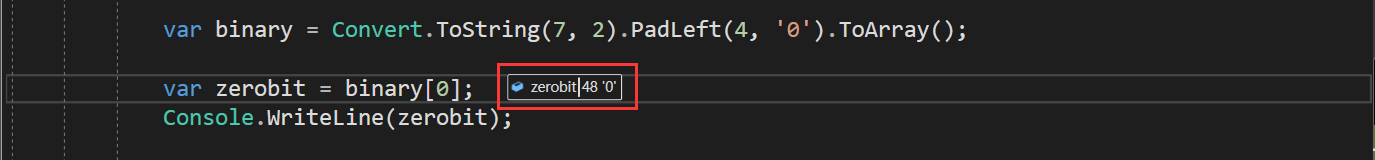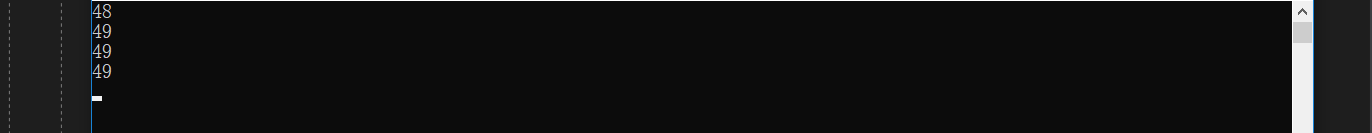C#由转换二进制所引起的思考，了解下？

二进制转换问题

var binary = Convert.ToString(7, 2).PadLeft(4, '0').ToArray();

var zerobit = binary;
Console.WriteLine(zerobit);foreach (var b in System.Text.Encoding.UTF8.GetBytes(binary))
{
Console.WriteLine(b.ToString());
}var zerobit = binary;
Console.WriteLine(zerobit.ToString());

转换字符数组问题

var binary = Convert.ToString(7, 2).PadLeft(4, '0').ToArray();

var binary1 = Convert.ToString(7, 2).PadLeft(4, '0').ToCharArray();

public unsafe char[] ToCharArray()
{
if (Length == 0)
{
return Array.Empty<char>();
}
char[] array = new char[Length];
fixed (char* smem = &_firstChar)
{
fixed (char* dmem = &array)
{
wstrcpy(dmem, smem, Length);
}
}
return array;
}
internal unsafe static void wstrcpy(char* dmem, char* smem, int charCount)
{
Buffer.Memmove((byte*)dmem, (byte*)smem, (uint)(charCount * 2));
}

public static TSource[] ToArray<TSource>(this IEnumerable<TSource> source)
{
if (source == null)
{
throw Error.ArgumentNull("source");
}
return new Buffer<TSource>(source).ToArray();
}

internal Buffer(IEnumerable<TElement> source)
{
TElement[] array = null;
int num = 0;
ICollection<TElement> collection = source as ICollection<TElement>;
if (collection != null)
{
num = collection.Count;
if (num > 0)
{
array = new TElement[num];
collection.CopyTo(array, 0);
}
}
else
{
foreach (TElement item in source)
{
if (array == null)
{
array = new TElement;
}
else if (array.Length == num)
{
TElement[] array2 = new TElement[checked(num * 2)];
Array.Copy(array, 0, array2, 0, num);
array = array2;
}
array[num] = item;
num++;
}
}
items = array;
count = num;
}

public sealed class CharEnumerator : IEnumerator, ICloneable, IEnumerator<char>, IDisposable
{......}

总结

posted @ 2020-07-02 07:15  Jeffcky  阅读(787)  评论(4编辑  收藏  举报